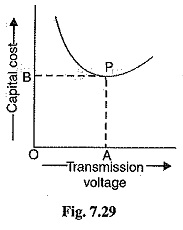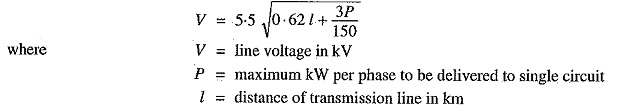## Economic Choice of Transmission Voltages:

It has been shown earlier here that if transmission voltage is increased, the volume of con­ductor material required is reduced. This decreases the expenditure on the conductor material. It may appear advisable to use the highest possible transmission voltage in order to reduce the expenditure on conductors to a minimum. However, it may be remembered that as the transmission voltages is increased, the cost of insulating the conductors, cost of transformers, switchgear and other terminal apparatus also increases. Therefore, for every transmission line, there is optimum transmission volt­ages, beyond which there is nothing to be gained in the matter of economy. The transmission voltages for which the cost of conductors, cost of insulators, transformers, switchgear and other terminal appa­ratus is minimum is called economical transmission voltage.

The method of finding the economical transmission voltage is as follows. Power to be transmit­ted, generation voltage and length of transmission line are assumed to be known. We choose some standard transmission voltages and work out the following costs :

• Transformers. at the generating and receiving ends of transmission line. For a given power, this cost increases slowly with the increase in transmission voltage.
• Switchgear. This cost also increases with the increase in transmission voltage.
• Lightning arrestor. This cost increases rapidly with the increase in transmission voltage.
• Insulation and supports. This cost increases sharply with the increase in transmission voltage.
• Conductor. This cost decreases with the increase in transmission voltage.The sum of all above costs gives the total cost of transmission for the voltage considered. Similar calculations are made for other transmission voltages. Then, a curve is drawn for total cost of transmission against voltage as shown in Fig. 7.29. The lowest point (P) on the curve gives the economical transmission voltage. Thus, in the present case, OA is the   optimum transmission voltages. This method of finding the economical transmission voltage is rarely used in practice as different costs cannot be determined with a fair degree of accuracy.

The present day trend is to follow certain empirical formulae for find­ing the economical transmission voltage. Thus, according to American practice, the economic voltage between lines in a 3-phase a.c. system isIt may be noted here that in the above formula, power to be transmitted and distance of transmis­sion line have been taken into account. It is because both these factors influence the economic voltage of a transmission line. This can be easily explained. If the distance of transmission line is increased, the cost of terminal apparatus is decreased, resulting in higher economic transmission voltages. Also if power to be transmitted in large, large generating and transforming units can be employed. This reduces the cost per kW of the terminal station equipment.

Scroll to Top